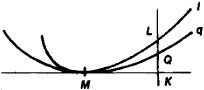# Osculation

Also found in: Dictionary, Thesaurus, Medical, Wikipedia.

## Osculation

The osculation of a curve q with a curve l at a given point M is a geometric concept meaning that q at M has contact with l of maximum order by comparison with the other curves in some preassigned family of curves {q} containing q.Figure 1

The order of contact of q and l is n if the length of the segment QL is an infinitesimal of order n + 1 relative to the length of the segment MK (see Figure 1). Here, QL is perpendicular, when extended, to the common tangent of q and l at M. Thus, with respect to the curves in {q}, the curve that is closest to l—that is, the curve for which the length of QL is an infinitesimal of maximum order—is the osculating curve of l at M. For example, the osculating circle of l at M is the circle whose order of contact with l is greater than that of any other circle.

The osculation of a surface q belonging to a given family of surfaces {q} with some curve / or with some surface at the point M of the curve or surface can be defined in much the same way. The order of contact here is defined in a manner similar to the above. We need only replace the tangent line MK shown in Figure 1 by the tangent plane of q at M.

### REFERENCES

La Vallée Poussin, C.-J. de. Kurs analiza beskonechno malykh, vol. 2. Leningrad-Moscow, 1933. (Translated from French.)
Il’in, V. A., and E. G. Pozniak. Osnovy matematicheskogo analiza, 3rd ed., part 1. Moscow, 1971.
References in periodicals archive ?
By modifying the osculation of the both raceways, one can obtain the decrease of the power losses of 10.20% of total power losses.
Of the latter, Kynaston McShine wrote, "Reducing Marilyn to an anatomical fragment, to a kind of repeating osculation machine, it dispels any romantic illusions about the idea of the kiss."
The "persisting, adhesive osculation"(5) of "prolonged pasturing on each other's lips ...
of murder by massive osculation, wherein a faithless lover is kissed
It's easy for non-fetishists to sneer about Pavlovian conditioning and let it go at that, but any underwear enthusiast worth his unwholesome giggle can tell you there is much more here--there is a cosmology: of nodes and cusps and points of osculation, mathematical kisses ...

Site: Follow: Share:
Open / Close